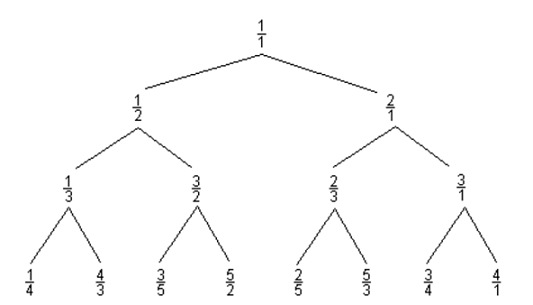TL;DR

On with TASK #2 from The Weekly Challenge #146. Enjoy!

# The challenge

Consider the following Curious Fraction Tree:You are given a fraction, member of the tree created similar to the above sample.

Write a script to find out the parent and grandparent of the given member.

Example 1:

Input: $member = '3/5'; Output: parent = '3/2' and grandparent = '1/2'  Example 2: Input:$member = '4/3';
Output: parent = '1/3' and grandparent = '1/2'


# The questions

This seems one of those induction-based challenges that can probably give rise to a whole host of wildly different interpretations and solutions. I’ll assume my take is… the right one (also in lack of alternatives, right now).

# The solution

From the example image, it seems that the parent of a valid node can be found as follows:

• every fraction has a numerator and a denominator
• if the numerator is smaller than the denominator, the parent shares the same numerator but the denominator is the difference between the denominator and the numerator from the child;
• otherwise, the parent’s numerator is the difference between the two, and the denominator is the same as the child.

#!/usr/bin/env perl
use v5.24;
use warnings;
use experimental 'signatures';
no warnings 'experimental::signatures';

my $member = shift // '4/3'; my$parent = parent_of($member); my$grandparent = parent_of($parent); say "parent = '$parent' and grandparent = '$grandparent'"; sub parent_of ($frac) {
my ($num,$den) = split m{/}mxs, $frac; join '/',$num < $den ? ($num, $den -$num) : ($num -$den, $den); }  Raku now: #!/usr/bin/env raku use v6; sub MAIN (Str:D$member = '4/3') {
my $parent = parent-of($member);
my $grandparent = parent-of($parent);
put "parent = '$parent' and grandparent = '$grandparent'";
}

sub parent-of ($frac) { my ($n, $d) =$frac.split: '/';
($n <$d ?? ($n,$d - $n) !! ($n - $d,$d)).join: '/';
}


It’s basically the same code, just a bit Raku-ized.

So… I hope I did read the challenge right! Anyway, stay safe everybody!